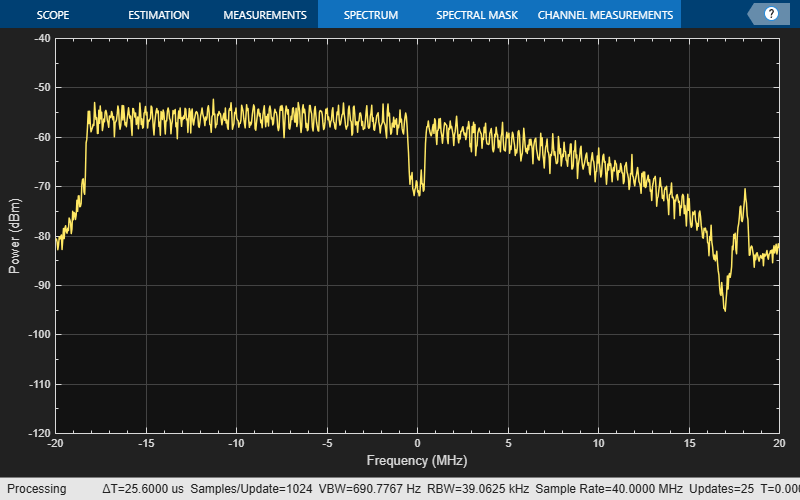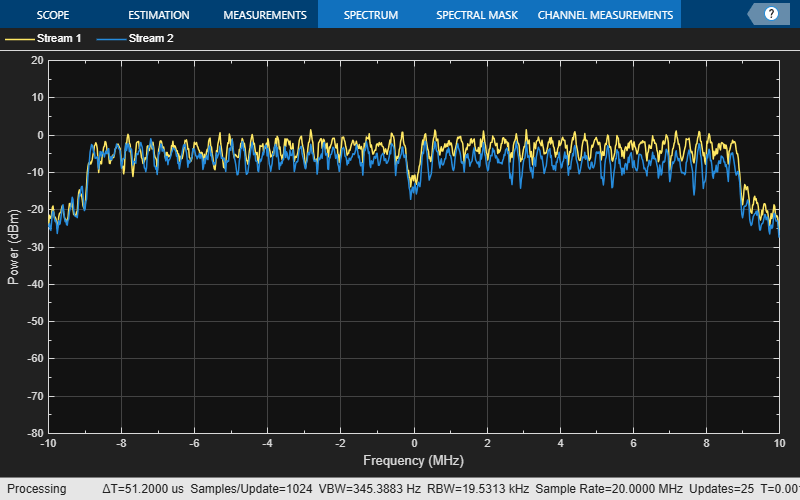Documentation

# wlanTGnChannel

Filter signal through 802.11n multipath fading channel

## Description

The `wlanTGnChannel` System object™ filters an input signal through an 802.11n™ (TGn) multipath fading channel.

The fading processing assumes the same parameters for all NT-by-NR links of the TGn channel. NT is the number of transmit antennas and NR is the number of receive antennas. Each link comprises all multipaths for that link.

To filter an input signal using a TGn multipath fading channel:

1. Create the `wlanTGnChannel` object and set its properties.

2. Call the object with arguments, as if it were a function.

## Creation

### Syntax

``tgn = wlanTGnChannel``
``tgn = wlanTGnChannel(Name,Value)``

### Description

example

````tgn = wlanTGnChannel` creates a TGn fading channel System object, `tgn`. This object filters a real or complex input signal through the TGn channel to obtain the channel-impaired signal.```
````tgn = wlanTGnChannel(Name,Value)` creates a TGn channel object, `tgn`, and sets properties using one or more name-value pairs. Enclose each property name in quotes. For example, `wlanTGnChannel('NumReceiveAntennas',2,'SampleRate',10e6)` creates a TGn channel with two receive antennas and a 10 MHz sample rate.```

## Properties

expand all

Unless otherwise indicated, properties are nontunable, which means you cannot change their values after calling the object. Objects lock when you call them, and the `release` function unlocks them.

If a property is tunable, you can change its value at any time.

Sample rate of the input signal in Hz, specified as a real positive scalar.

Data Types: `double`

Delay profile model, specified as `'Model-A'`, `'Model-B'`, `'Model-C'`, `'Model-D'`, `'Model-E'`, or `'Model-F'`.

The table summarizes the models properties before the bandwidth reduction factor.

ParameterModel
ABCDEF
Breakpoint distance (m)555102030
Maximum delay (ns)0802003907301050
Rician K-factor (dB)000366
Number of clusters122346
Number of taps1914181818

Data Types: `char` | `string`

RF carrier frequency in Hz, specified as a real positive scalar.

Data Types: `double`

Speed of the scatterers in km/h, specified as a real positive scalar.

Data Types: `double`

Distance between the transmitter and receiver in meters, specified as a real positive scalar.

`TransmitReceiveDistance` is used to compute the path loss, and to determine whether the channel has a line of sight (LOS) or non line of sight (NLOS) condition. The path loss and standard deviation of shadow fading loss depend on the separation between the transmitter and the receiver.

Data Types: `double`

Normalize path gains, specified as `true` or `false`. To normalize the fading processes such that the total power of the path gains, averaged over time, is 0 dB, set this property to `true` (default). When you set this property to `false`, the path gains are not normalized.

Data Types: `logical`

Number of transmit antennas, specified as a positive integer from `1` to `4`.

Data Types: `double`

Distance between transmit antenna elements, specified as a real positive scalar expressed in wavelengths.

`TransmitAntennaSpacing` supports uniform linear arrays only.

#### Dependencies

This property applies only when `NumTransmitAntennas` is greater than `1`.

Data Types: `double`

Number of receive antennas, specified as a positive integer from 1 to 4.

Data Types: `double`

Distance between receive antenna elements, specified as a real positive scalar expressed in wavelengths.

`ReceiveAntennaSpacing` supports uniform linear arrays only.

#### Dependencies

This property applies only when `NumReceiveAntennas` is greater than `1`.

Data Types: `double`

Large-scale fading effects applied in the channel, specified as `'None'`, `'Pathloss'`, `'Shadowing'`, or `'Pathloss and shadowing'`.

Data Types: `char` | `string`

Fluorescent effect, specified as `true` or `false`. To include Doppler effects from fluorescent lighting set this property to `true`.

#### Dependencies

The `FluorescentEffect` property applies only when `DelayProfile` is `'Model-D'` or `'Model-E'`.

Data Types: `logical`

Power line frequency in Hz, specified as `'50Hz'` or `'60Hz'`.

The power line frequency is 60 Hz in the United States and 50 Hz in Europe.

#### Dependencies

This property applies only when you set `FluorescentEffect` to `true` and `DelayProfile` to `'Model-D'` or `'Model-E'`.

Data Types: `char` | `string`

Normalize channel outputs by the number of receive antennas, specified as a `true` or `false`.

Data Types: `logical`

Enable channel filtering, specified as a logical value of `true` or `false`. To enable channel filtering, set this property to `true`. To disable channel filtering, set this property to `false`.

### Note

If you set this property to `false`, the `step` object function does not accept an input signal. In this case, the `NumSamples` and `SampleRate` properties determine the duration of the fading process realization.

Data Types: `logical`

Number of time-domain samples used to get path gain samples, specified as a positive integer.

#### Dependencies

To enable this property, set the `ChannelFiltering` property to `false`.

Data Types: `double`

Source of random number stream, specified as `'Global stream'` or `'mt19937ar with seed'`.

If you set `RandomStream` to `'Global stream'`, the current global random number stream generates normally distributed random numbers. In this case, the `reset` function resets the filters only.

If you set `RandomStream` to ```'mt19937ar with seed'```, the mt19937ar algorithm generates normally distributed random numbers. In this case, the `reset` function also reinitializes the random number stream to the value of the `Seed` property.

Data Types: `char` | `string`

Initial seed of an mt19937ar random number stream, specified as a nonnegative integer. The `Seed` property reinitializes the mt19937ar random number stream in the `reset` function.

#### Dependencies

This property applies only when you set the `RandomStream` property to `'mt19937ar with seed'`.

Data Types: `double`

Enable path gain output computation, specified as `true` or `false`.

Data Types: `logical`

## Usage

### Syntax

``y = tgn(x)``
``[y,pathGains] = tgn(x)``

### Description

example

````y = tgn(x)` filters input signal `x` through the TGn fading channel defined by the `wlanTGnChannel` System object, `tgn`, and returns the result in `y`.```
````[y,pathGains] = tgn(x)` also returns in `pathGains` the TGn channel path gains of the underlying fading process. This syntax applies when you set the `PathGainsOutputPort` property to `true`.```

### Input Arguments

expand all

Input signal, specified as a real or complex NS-by-NT matrix, where:

• NS is the number of samples.

• NT is the number of transmit antennas and must be equal to the `NumTransmitAntennas` property value.

Data Types: `double`
Complex Number Support: Yes

### Output Arguments

expand all

Output signal, returned as an NS-by-NR complex matrix, where:

• NS is the number of samples.

• NR is the number of receive antennas and is equal to the `NumReceiveAntennas` property value.

Data Types: `double`

Path gains of the fading process, returned as an NS-by-NP-by-NT-by-NR complex array, where:

• NS is the number of samples.

• NP is the number of resolvable paths, that is, the number of paths defined for the case specified by the `DelayProfile` property.

• NT is the number of transmit antennas and is equal to the `NumTransmitAntennas` property value.

• NR is the number of receive antennas and is equal to the `NumReceiveAntennas` property value.

Data Types: `double`

## Object Functions

To use an object function, specify the System object as the first input argument. For example, to release system resources of a System object named `obj`, use this syntax:

`release(obj)`

expand all

 `info` Characteristic information about TGn, TGah, TGac, and TGax multipath fading channels
 `step` Run System object algorithm `release` Release resources and allow changes to System object property values and input characteristics `reset` Reset internal states of System object

### Note

`reset`: If the `RandomStream` property of the System object is set to `'Global stream'`, the `reset` function resets the filters only. If you set `RandomStream` to `'mt19937ar with seed'`, the `reset` function also reinitializes the random number stream to the value of the `Seed` property.

## Examples

expand all

Generate an HT waveform and pass it through a TGn SISO channel. Display the spectrum of the resultant signal.

Set the channel bandwidth and the corresponding sample rate.

```bw = 'CBW40'; fs = 40e6; ```

Generate an HT waveform for a 40 MHz channel.

```cfg = wlanHTConfig('ChannelBandwidth',bw); txSig = wlanWaveformGenerator(randi([0 1],1000,1),cfg); ```

Create a TGn SISO channel with path loss and shadowing enabled.

```tgnChan = wlanTGnChannel('SampleRate',fs, ... 'LargeScaleFadingEffect','Pathloss and shadowing'); ```

Pass the HT waveform through the channel.

```rxSig = tgnChan(txSig); ```

Plot the spectrum of the received waveform.

```saScope = dsp.SpectrumAnalyzer('SampleRate',fs,'YLimits',[-120 -40]); saScope(rxSig) ```Because path loss and shadowing are enabled, the mean received power across the spectrum is approximately -60 dBm.

Create an HT waveform having four transmit antennas and two space-time streams.

```cfg = wlanHTConfig('NumTransmitAntennas',4,'NumSpaceTimeStreams',2, ... 'SpatialMapping','Fourier'); txSig = wlanWaveformGenerator([1;0;0;1],cfg); ```

Create a 4x2 MIMO TGn channel and disable large-scale fading effects.

```tgnChan = wlanTGnChannel('SampleRate',20e6, ... 'NumTransmitAntennas',4, ... 'NumReceiveAntennas',2, ... 'LargeScaleFadingEffect','None'); ```

Pass the transmit waveform through the channel.

```rxSig = tgnChan(txSig); ```

Display the spectrum of the two received space-time streams.

```saScope = dsp.SpectrumAnalyzer('SampleRate',20e6, ... 'ShowLegend',true, ... 'ChannelNames',{'Stream 1','Stream 2'}); saScope(rxSig) ```Transmit an HT-LTF and an HT data field through a noisy 2x2 MIMO channel. Demodulate the received HT-LTF to estimate the channel coefficients. Recover the HT data and determine the number of bit errors.

Set the channel bandwidth and corresponding sample rate.

```bw = 'CBW40'; fs = 40e6;```

Create HT-LTF and HT data fields having two transmit antennas and two space-time streams.

```cfg = wlanHTConfig('ChannelBandwidth',bw, ... 'NumTransmitAntennas',2,'NumSpaceTimeStreams',2); txPSDU = randi([0 1],8*cfg.PSDULength,1); txLTF = wlanHTLTF(cfg); txDataSig = wlanHTData(txPSDU,cfg);```

Create a 2x2 MIMO TGn channel with path loss and shadowing enabled.

```tgnChan = wlanTGnChannel('SampleRate',fs, ... 'NumTransmitAntennas',2,'NumReceiveAntennas',2, ... 'LargeScaleFadingEffect','None');```

Create AWGN channel noise, setting SNR = 15 dB.

```chNoise = comm.AWGNChannel('NoiseMethod','Signal to noise ratio (SNR)',... 'SNR',15);```

Pass the signals through the TGn channel and noise models.

```rxLTF = chNoise(tgnChan(txLTF)); rxDataSig = chNoise(tgnChan(txDataSig));```

Create an AWGN channel for a 40 MHz channel with a 9 dB noise figure. The noise variance, `nVar`, is equal to kTBF, where k is Boltzmann's constant, T is the ambient temperature of 290 K, B is the bandwidth (sample rate), and F is the receiver noise figure.

```nVar = 10^((-228.6 + 10*log10(290) + 10*log10(fs) + 9)/10); awgnChan = comm.AWGNChannel('NoiseMethod','Variance','Variance',nVar);```

Pass the signals through the channel.

```rxLTF = awgnChan(rxLTF); rxDataSig = awgnChan(rxDataSig);```

Demodulate the HT-LTF. Use the demodulated signal to estimate the channel coefficients.

```dLTF = wlanHTLTFDemodulate(rxLTF,cfg); chEst = wlanHTLTFChannelEstimate(dLTF,cfg);```

Recover the data and determine the number of bit errors.

```rxPSDU = wlanHTDataRecover(rxDataSig,chEst,nVar,cfg); numErr = biterr(txPSDU,rxPSDU)```
```numErr = 0 ```

## Algorithms

expand all

The 802.11n channel object uses a filtered Gaussian noise model in which the path delays, powers, angular spread, angles of arrival, and angles of departure are determined empirically. The specific modeling approach is described in .

 Erceg, V., L. Schumacher, P. Kyritsi, et al. TGn Channel Models. Version 4. IEEE 802.11-03/940r4, May 2004.

 Kermoal, J. P., L. Schumacher, K. I. Pedersen, P. E. Mogensen, and F. Frederiksen, “A Stochastic MIMO Radio Channel Model with Experimental Validation”. IEEE Journal on Selected Areas in Communications., Vol. 20, No. 6, August 2002, pp. 1211–1226.

##### SupportGet trial now